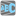• # what is wrong with assert statement,

## Question related to missionThree Words

def checkio(words: str) -> bool: count = 0 mylst = list(words.split())

```if len(words) < 3:
return False

for w in mylst:
#print(f'== {w} == letter: { w.isalpha()} a num: {w.isdigit() } n count {count}')
if w.isalpha():
count += 1
elif w.isdigit() and w.isalnum():
count = 0 # matched a num, start the count over! This is how we count the required 3 consecutive words.
if count >= 3: # when we hit 3, return True
return True
return False
```

# These "asserts" using only for self-checking and not necessary for auto-testing

if name == 'main': print('Example:') print(checkio("Hello World hello")) print( checkio("one two 3 four five 6 seven eight 9 ten eleven 12")) print('-'*15)

```assert checkio("He is 123 man") == False, "123 man" # assert only returns a bool, so str can be empty "" I think?
assert checkio("Hello World hello") == True, "Hello World hello"
assert checkio("He is 123 man") == False, "123 man"
assert checkio("He is 123 man") == False, "123 man" # assert only returns a bool, so str can be empty "" I think?
assert checkio("1 2 3 4") == False, "Digits"
assert checkio("bla bla bla bla") == True, "Bla Bla"
assert checkio("Hi") == False, "Hi"
assert checkio("one two 3 four five six 7 eight 9 ten eleven 12") == True, "four five six"  # <- why is this wrong? HELP! I SPENT ALL NIGHT ON THIS!
print("Coding complete? Click 'Check' to review your tests and earn cool rewards!")
```7